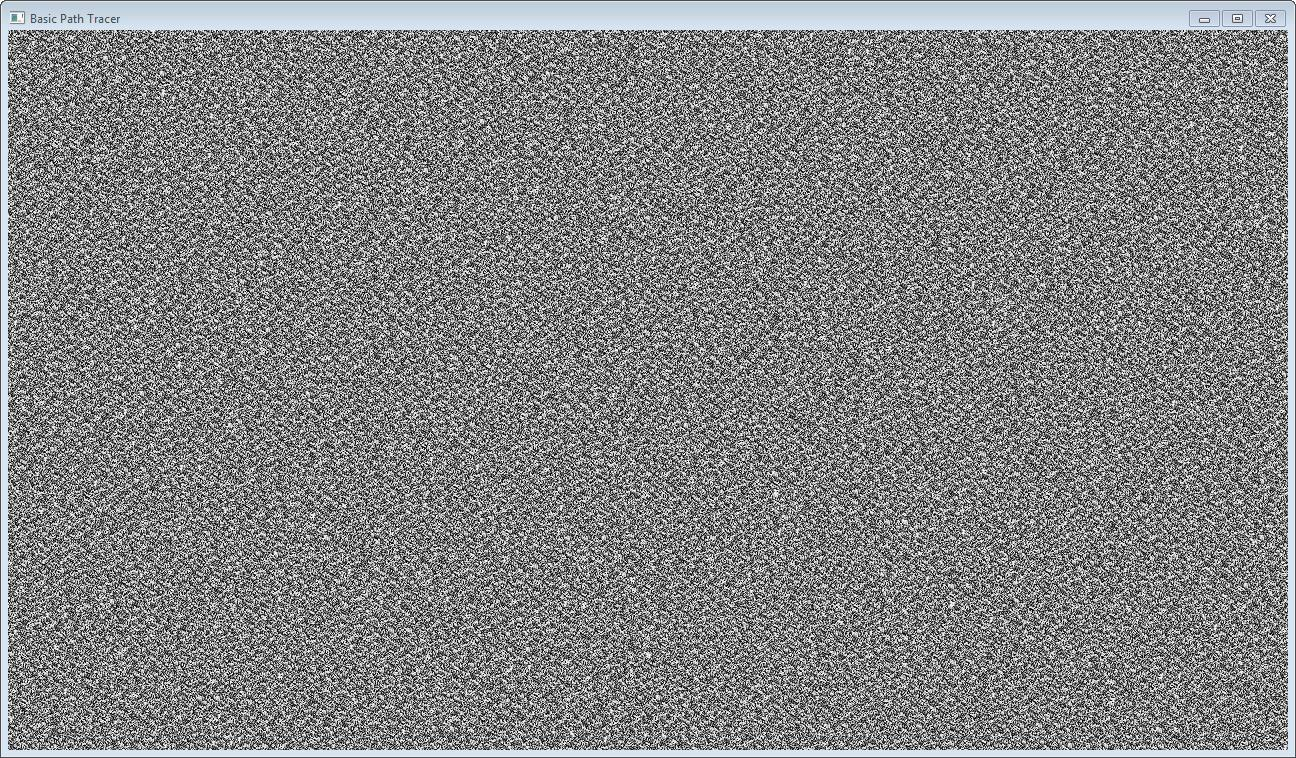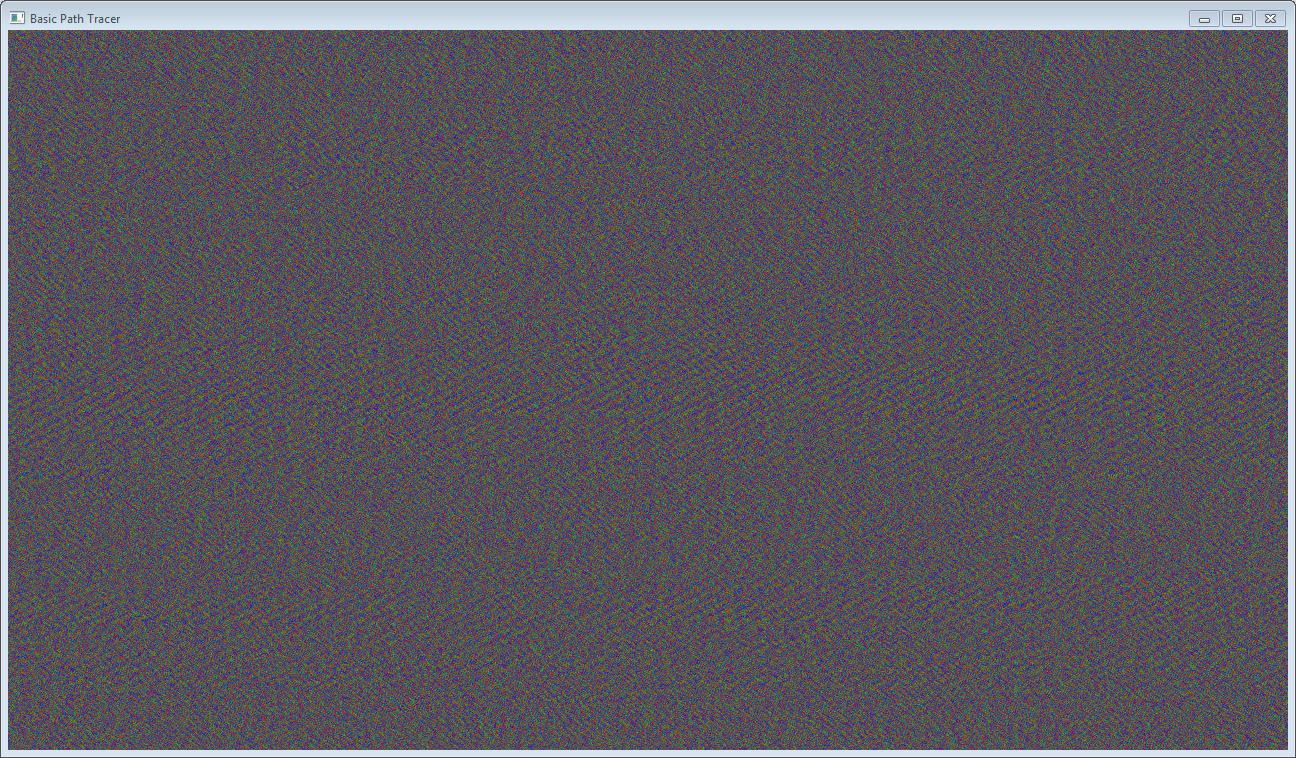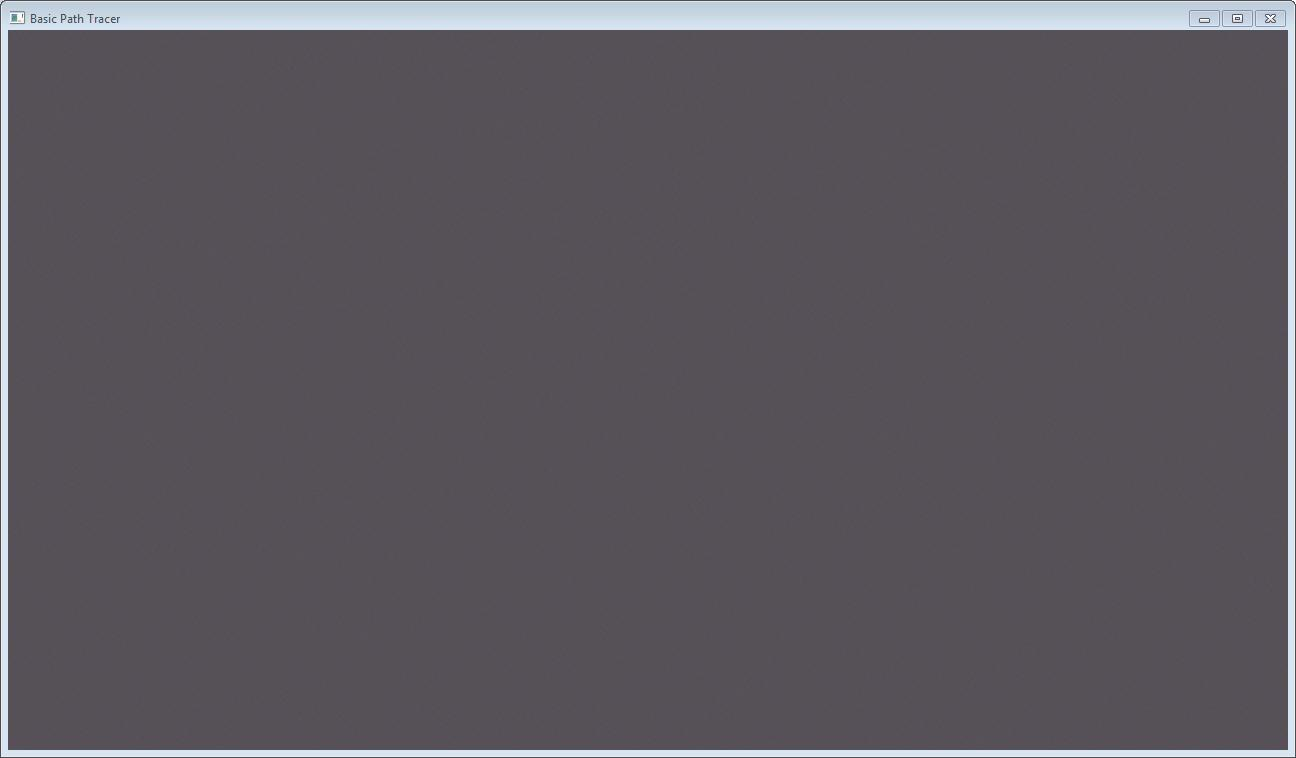### Creating Randomness and Acummulating Change

This is the second post in a series documenting my adventures in creating a GPU path tracer from scratch. If you missed it, the first post is here.

Path tracing uses Monte Carlo Integration to estimate the Global Illumination in the scene. In our case, Monte Carlo Integration boils down to taking a large number of random samples of the scene, and averaging them together. Random is the key word here. If we don’t randomly sample, the resulting image will have artifacts in the form of patterns, banding, etc.

### Creating Randomness from the Non-Random

So how do we create random numbers? This is a really old topic that has been extensively researched, so rather than reiterate it here, I’ll just point you to Google. The point we do care about, though, is where we create the random numbers. As far as I can see, our options are as follows:

1. Generate a large number of random numbers on the CPU using classic psuedo-random number generators (PRNG), and transfer them to the GPU to be consumed as needed.
2. Create a random number generator on the GPU, and access it from each thread
3. Create a random number generator per thread on the GPU

While option 1 looks simple and straightforward, path tracing will use a large number of random numbers, and each number consumed has to be transferred across the bus from the CPU to the GPU. This is going to be SLOW.

Ok, with the CPU out of the picture, we need to find a PRNG for the GPU. Luckily, CUDA comes with a library that does just that: curand. But, how many PRNGs should we have, and where should they live?

To better understand the problem, let’s briefly go over how PRNGs work. PRNGs use math to simulate random sequences. In order to get different numbers, they need to access and store internal state, aka data. The size of this state depends on the PRNG algorithm.

If we choose option 2, every single thread will want access to a single generator. There will be massive contention, and random number generation will degrade to a serial operation. Again, since path tracing requires a large number of random numbers, this option will be slow.

So option 3 it is! It turns out that the size of the state for the default curand generator isn’t that big, so storing state per thread isn’t too bad.

### Implementing curand in the Kernel

In order to create a generator in the kernel, you have to create a curandstate object and then call curand_init() on it, passing in a seed, a sequence number, and an offset.

curandState randState;
curand_init(seed, sequenceNum, offset, &randState);


Then, you can generate random numbers using:

uint64 randInteger = curand(&randState);
float randNormalizedFloat = curand_uniform(&randState);


Two states with different seeds will create a different sequence of random numbers. Two states with the same seed will create the same sequence of random numbers.

Two states with the same seed, but different sequenceNum will use the same sequence, but be offset to different blocks of the sequence. (Specifically, in increments of 267) Why would you want this? According to the documentation, “Sequences generated with the same seed and different sequence numbers will not have statistically correlated values.”

Offset just manually skips ahead n in the sequence.

In the curand documentation, the creators mention that curand_init() can be relatively slow, so if you’re launching the same kernel multiple times, it’s usually better to keep one curandstate per thread, but to store it in global memory between kernel launches. ie:

__global__ void setupRandStates(curandState *state) {
int id = threadIdx.x + blockIdx.x * blockDim.x;

// Each thread gets same seed, a different sequence number, no offset
curand_init(1234, id, 0, &state[id]);
}

__global__ void useRandStates(curandState *state) {
int id = threadIdx.x + blockIdx.x * blockDim.x;

// Copy state to local memory for efficiency
curandState localState = state[id];

// Use localState to generate numbers....

// Copy state back to global memory
state[id] = localState;
}

int main() {
int numBlocks = 64;

// Allocate space on the device to store the random states
curandState *d_randStates;
cudaMalloc(&d_randStates, numBlocks * numThreads * sizeof(curandState));

// Setup and use the randStates

for (uint i = 0; i < NUM_ITERATIONS; ++i) {
}

return 0;
}


This would be really nice, but there is one problem: storing all the states:

cudaMalloc(&d_randStates, numBlocks * numThreads * sizeof(curandState));


In our case, we’ll be launching a thread for every pixel on the screen. aka, millions of threads. While curandState isn’t that large, storing millions of them is not feasible. So what can we do instead? It turns out that curand_init() is only slow if you use sequenceNum and offset. This is quite intuitive, since using those requires the generator to skip ahead a large amount. So if we keep both sequenceNum and offset equal to zero, curand_init() is quite fast.

In order to give each thread different random numbers we give them unique seeds. A simple method I came up with is to hash the frameNumber and then add the id of thread.

uint32 WangHash(uint32 a) {
a = (a ^ 61) ^ (a >> 16);
a = a + (a << 3);
a = a ^ (a >> 4);
a = a * 0x27d4eb2d;
a = a ^ (a >> 15);
return a;
}

int threadId = (blockIdx.x + blockIdx.y * gridDim.x) * (blockDim.x * blockDim.y) + (threadIdx.y * blockDim.x) + threadIdx.x;

// Create random number generator
curandState randState;

// Use randState to generate numbers...
}

int main() {
uint width = 1024;
uint height = 256;

dim3 Db = dim3(16, 16);   // block dimensions are fixed to be 256 threads
dim3 Dg = dim3((width + Db.x - 1) / Db.x, (height + Db.y - 1) / Db.y);

for (uint i = 0; i < NUM_ITERATIONS; ++i) {
}

return 0;
}


Yay! Now we can generate lots of random numbers! If we generate a random number on each thread and output it as a greyscale color, we can make some nice white noise.### Accumulating and Averaging Colors

The last part of Monte Carlo Integration is the averaging of all the samples taken. The simplest solution is to just accumulate the colors from each frame, adding one frame to the next. Then, at the end, we divide the color at each pixel by the number of frames. I integrated this into my code by passing the frame number into the pixel shader that draws the texture to the screen.

cbuffer constants {
float gInverseNumPasses;
};

Texture2D<float3> gHDRInput : register(t0);

float4 CopyCudaOutputToBackbufferPS(CalculatedTrianglePixelIn input) : SV_TARGET {
return float4(gHDRInput[input.positionClip.xy] * gInverseNumPasses, 1.0f);
}


This way, I can see the accumulated output as it’s being generated. If we create a simple kernel that outputs either pure red, green, or blue, depending on a random number, we can test if the accumulation buffer is working.

__global__ void AccumulationBufferTest(unsigned char *textureData, uint width, uint height, size_t pitch, DeviceCamera camera, uint hashedFrameNumber) {
int x = blockIdx.x * blockDim.x + threadIdx.x;
int y = blockIdx.y * blockDim.y + threadIdx.y;

if (x >= width || y >= height) {
return;
}

int threadId = (blockIdx.x + blockIdx.y * gridDim.x) * (blockDim.x * blockDim.y) + (threadIdx.y * blockDim.x) + threadIdx.x;

// Create random number generator
curandState randState;

// Generate a uniform random number
float randNum = curand_uniform(&randState);

// Get a pointer to the pixel at (x,y)
float *pixel = (float *)(textureData + y * pitch) + 4 /*RGBA*/ * x;

if (x < width && y < height) {
// Write out pixel data
if (randNum < 0.33f) {
pixel += 1.0f;
pixel += 0.0f;
pixel += 0.0f;
pixel = 1.0f;
} else if (randNum < 0.66f) {
pixel += 0.0f;
pixel += 1.0f;
pixel += 0.0f;
pixel = 1.0f;
} else {
pixel += 0.0f;
pixel += 0.0f;
pixel += 1.0f;
pixel = 1.0f;
}
}
}


After the first 60 frames, the image is quite noisy:However, if we let it sit for a bit, the image converges to the a gray (0.33, 0.33, 0.33) as expected:### Conclusion

Well, there we go! We can generate random numbers and average the results from several frames. The next post will cover ray-object intersections and maybe start in on path tracing itself. Stay tuned!

The code for everything in this post is on GitHub. It’s open source under Apache license, so feel free to use it in your own projects.

As always, feel free to ask questions, make comments, and if you find an error, please let me know.

Happy coding!